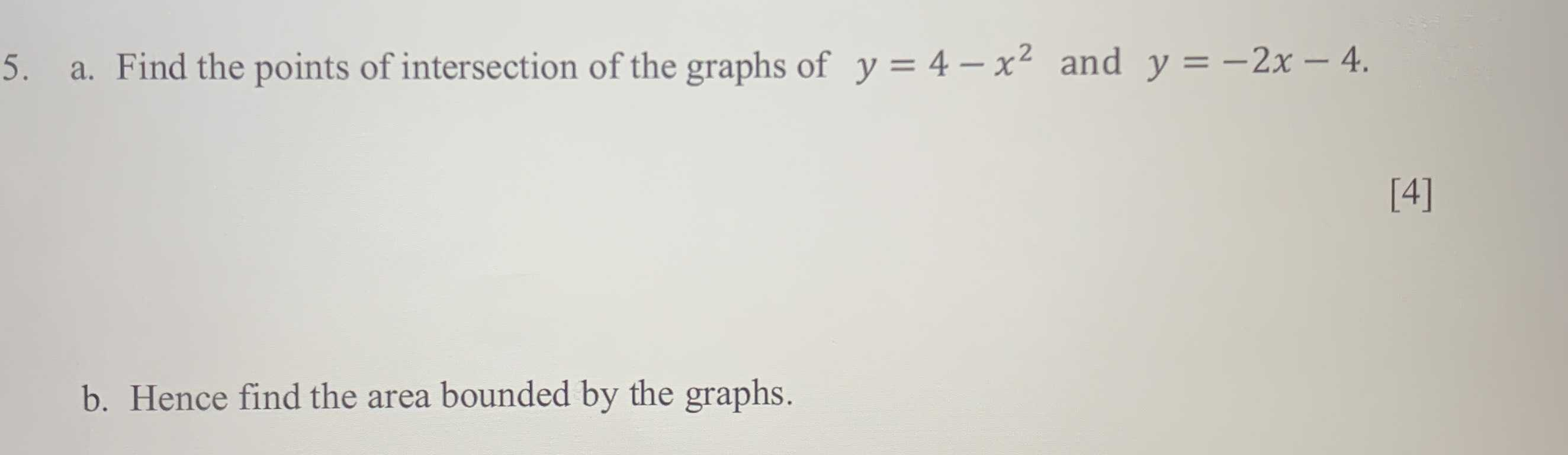### ¿Todavía tienes preguntas de matemáticas?

Pregunte a nuestros tutores expertos
Algebra
Pregunta5. a. Find the points of intersection of the graphs of $$y = 4 - x ^ { 2 }$$ and $$y = - 2 x - 4$$ .

b. Hence find the area bounded by the graphs.